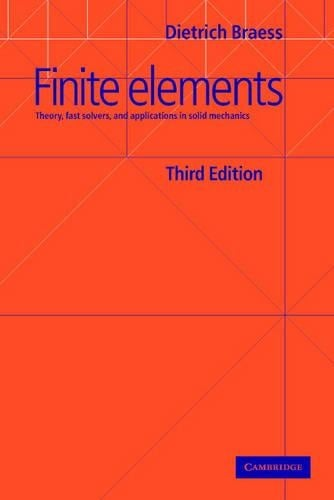•# Finite Elements: Theory, Fast Solvers, and Applications in Solid Mechanics (Paperback)

(author)
£47.99
Paperback 384 Pages / Published: 12/04/2007
• We can order this

Usually dispatched within 3 weeks

This definitive introduction to finite element methods was thoroughly updated for this 2007 third edition, which features important material for both research and application of the finite element method. The discussion of saddle-point problems is a highlight of the book and has been elaborated to include many more nonstandard applications. The chapter on applications in elasticity now contains a complete discussion of locking phenomena. The numerical solution of elliptic partial differential equations is an important application of finite elements and the author discusses this subject comprehensively. These equations are treated as variational problems for which the Sobolev spaces are the right framework. Graduate students who do not necessarily have any particular background in differential equations, but require an introduction to finite element methods will find this text invaluable. Specifically, the chapter on finite elements in solid mechanics provides a bridge between mathematics and engineering.

Publisher: Cambridge University Press
ISBN: 9780521705189
Number of pages: 384
Weight: 560 g
Dimensions: 229 x 152 x 22 mm
Edition: 3rd Revised edition

MEDIA REVIEWS
'This is a well written book on the mathematical foundation of the finite element method which should appeal to graduate mathematicians and researchers in numerical methods and theoretical mechanics. The coverage of the mathematical theories used in the finite element formulation is comprehensive.' A. A. Becker, Journal of Mechanical Engineering Science
'... an excellent book on the subject, with respect to contents, readability as well as presentation ... highly recommended.' Michael Schafer, Zentralblatt fur Mathematik
'... has advanced to be one of the most commonly used textbooks in this area.' Monatshefte fur Mathematik
'Carefully written and remarkably error-free, Braess's book introduces partial differential equations (PDEs) and methods used to solve them numerically. It introduces PDEs and their classification, covers (briefly) finite-difference methods, and then offers a thorough treatment of finite-element methods, both conforming and nonconforming. After discussing the conjugate gradient method and multigrid methods, Braess concludes with a chapter on finite elements in solid mechanics. The book is written from a theoretical standpoint, and the standard convergence theorems and error estimates are provided and proved. Although a background in differential equations, analysis, and linear algebra is not necessary to read the book, it would be helpful. The level is that of a graduate course in a mathematics department. Practical considerations for coding the various methods are only occasionally discussed. There are exercises at the end of each section varying from two to six problems, about two-thirds of them theoretical in nature. The book can be used as a resource. Extensive and valuable bibliography. Recommended for graduate students.' J. H. Ellison, Grove City College